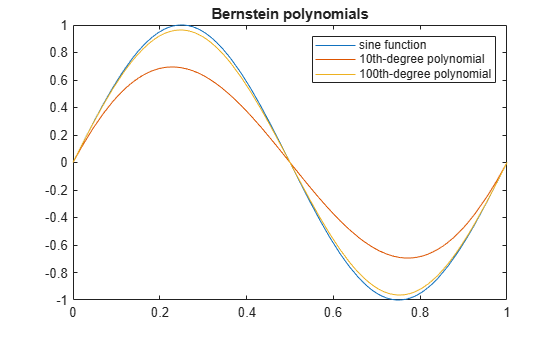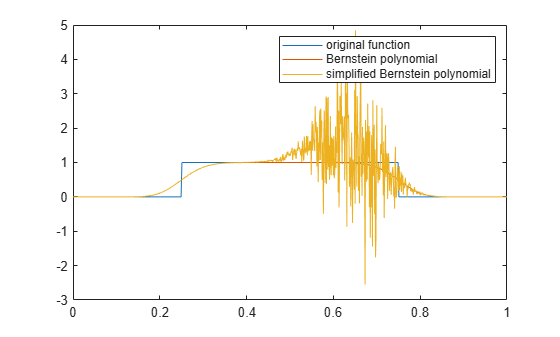Main Content

# bernstein

Bernstein polynomials

## Syntax

``bernstein(f,n,t)``
``bernstein(g,n,t)``
``bernstein(g,var,n,t)``

## Description

example

````bernstein(f,n,t)` with a function handle `f` returns the `n`th-order Bernstein polynomial `symsum(nchoosek(n,k)*t^k*(1-t)^(n-k)*f(k/n),k,0,n)`, evaluated at the point `t`. This polynomial approximates the function `f` over the interval `[0,1]`.```

example

````bernstein(g,n,t)` with a symbolic expression or function `g` returns the `n`th-order Bernstein polynomial, evaluated at the point `t`. This syntax regards `g` as a univariate function of the variable determined by `symvar(g,1)`.If any argument is symbolic, `bernstein` converts all arguments except a function handle to symbolic, and converts a function handle’s results to symbolic.```

example

````bernstein(g,var,n,t)` with a symbolic expression or function `g` returns the approximating `n`th-order Bernstein polynomial, regarding `g` as a univariate function of the variable `var`.```

## Examples

### Approximation of Sine Function Specified as Function Handle

Approximate the sine function by the 10th- and 100th-degree Bernstein polynomials.

```syms t b10 = bernstein(@(t) sin(2*pi*t), 10, t); b100 = bernstein(@(t) sin(2*pi*t), 100, t);```

Plot `sin(2*pi*t)` and its approximations.

```fplot(sin(2*pi*t),[0,1]) hold on fplot(b10,[0,1]) fplot(b100,[0,1]) legend('sine function','10th-degree polynomial',... '100th-degree polynomial') title('Bernstein polynomials') hold off```### Approximation of Exponential Function Specified as Symbolic Expression

Approximate the exponential function by the second-order Bernstein polynomial in the variable `t`:

```syms x t bernstein(exp(x), 2, t)```
```ans = (t - 1)^2 + t^2*exp(1) - 2*t*exp(1/2)*(t - 1)```

Approximate the multivariate exponential function. When you approximate a multivariate function, `bernstein` regards it as a univariate function of the default variable determined by `symvar`. The default variable for the expression `y*exp(x*y)` is `x`:

```syms x y t symvar(y*exp(x*y), 1)```
```ans = x```

`bernstein` treats this expression as a univariate function of `x`:

`bernstein(y*exp(x*y), 2, t)`
```ans = y*(t - 1)^2 + t^2*y*exp(y) - 2*t*y*exp(y/2)*(t - 1)```

To treat `y*exp(x*y)` as a function of the variable `y`, specify the variable explicitly:

`bernstein(y*exp(x*y), y, 2, t)`
```ans = t^2*exp(x) - t*exp(x/2)*(t - 1)```

### Approximation of Linear Ramp Specified as Symbolic Function

Approximate function `f` representing a linear ramp by the fifth-order Bernstein polynomials in the variable `t`:

```syms f(t) f(t) = triangularPulse(1/4, 3/4, Inf, t); p = bernstein(f, 5, t)```
```p = 7*t^3*(t - 1)^2 - 3*t^2*(t - 1)^3 - 5*t^4*(t - 1) + t^5```

Simplify the result:

`simplify(p)`
```ans = -t^2*(2*t - 3)```

### Numerical Stability of Simplified Bernstein Polynomials

When you simplify a high-order symbolic Bernstein polynomial, the result often cannot be evaluated in a numerically stable way.

Approximate this rectangular pulse function by the 100th-degree Bernstein polynomial, and then simplify the result.

```f = @(x)rectangularPulse(1/4,3/4,x); b1 = bernstein(f, 100, sym('t')); b2 = simplify(b1);```

Convert the polynomial `b1` and the simplified polynomial `b2` to MATLAB® functions.

```f1 = matlabFunction(b1); f2 = matlabFunction(b2);```

Compare the plot of the original rectangular pulse function, its numerically stable Bernstein representation `f1`, and its simplified version `f2`. The simplified version is not numerically stable.

```t = 0:0.001:1; plot(t, f(t), t, f1(t), t, f2(t)) hold on legend('original function','Bernstein polynomial',... 'simplified Bernstein polynomial') hold off```## Input Arguments

collapse all

Function to be approximated by a polynomial, specified as a function handle. `f` must accept one scalar input argument and return a scalar value.

Function to be approximated by a polynomial, specified as a symbolic expression or function.

Bernstein polynomial order, specified as a nonnegative number.

Evaluation point, specified as a number, symbolic number, variable, expression, or function. If `t` is a symbolic function, the evaluation point is the mathematical expression that defines `t`. To extract the mathematical expression defining `t`, `bernstein` uses `formula(t)`.

Free variable, specified as a symbolic variable.

## More About

collapse all

### Bernstein Polynomials

A Bernstein polynomial is a linear combination of Bernstein basis polynomials.

A Bernstein polynomial of degree `n` is defined as follows:

`$B\left(t\right)=\sum _{k=0}^{n}{\beta }_{k}{b}_{k,n}\left(t\right).$`

Here,

are the Bernstein basis polynomials, and $\left(\begin{array}{c}n\\ k\end{array}\right)$ is a binomial coefficient.

The coefficients ${\beta }_{k}$ are called Bernstein coefficients or Bezier coefficients.

If `f` is a continuous function on the interval ```[0, 1]``` and

`${B}_{n}\left(f\right)\left(t\right)=\sum _{k=0}^{n}f\left(\frac{k}{n}\right){b}_{k,n}\left(t\right)$`

is the approximating Bernstein polynomial, then

`$\underset{n\to \text{ }\infty }{\mathrm{lim}}{B}_{n}\left(f\right)\left(t\right)=f\left(t\right)$`

uniformly in `t` on the interval ```[0, 1]```.

## Tips

• Symbolic polynomials returned for symbolic `t` are numerically stable when substituting numerical values between `0` and `1` for `t`.

• If you simplify a symbolic Bernstein polynomial, the result can be unstable when substituting numerical values for the curve parameter `t`.

## See Also

Introduced in R2013b

## Support

#### Mathematical Modeling with Symbolic Math Toolbox

Get examples and videos